Aishwarya Singh — Published On August 3, 2020 and Last Modified On August 4th, 2020

## Overview

• Understand the concept of Non-Max Suppression
• Learn how object detection algorithms use Non-Max Suppression
• Implement non-max suppression using NMS function in PyTorch

## Introduction

Computer vision is one of the most glaring fields in data science. Like any other field of data science, the applications of this field has also become a part of our personal lives. For example, image classification, pose estimation, object detection, etc are some of its applications and we are all surrounded by it. Refer to this article-

I was recently studying algorithms for object detection and I came across a very interesting idea that almost all of these algorithms use – Non-Max Suppression (or NMS).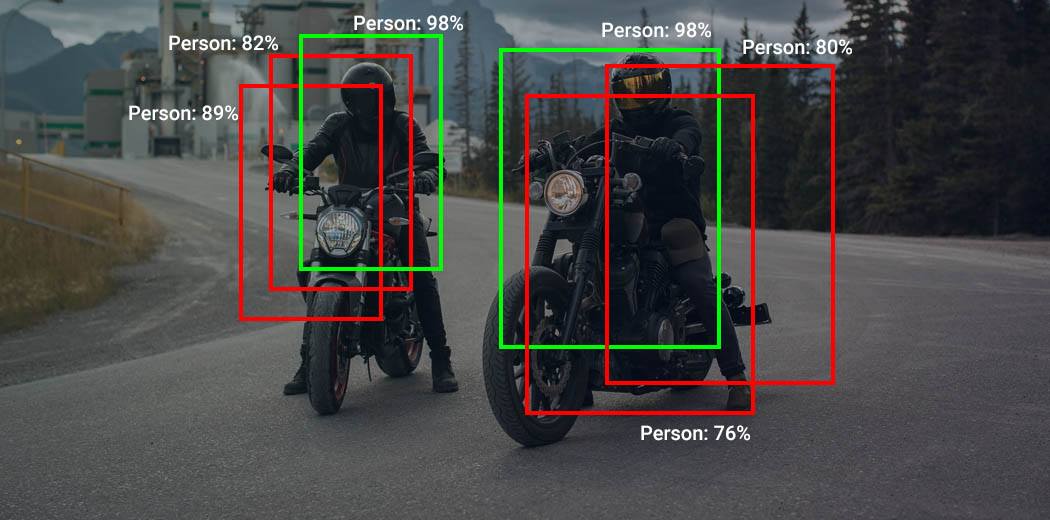Non-max suppression is the final step of these object detection algorithms and is used to select the most appropriate bounding box for the object.

In this article, I will introduce the concept of non-max suppression, why it is used, and explain how it works in the object detection algorithms.

1. Introduction to Object Detection
2. What is non-max suppression?
3. How does non-max suppression work?
4. Pseudo code for non-max suppression
5. Implementation of non-max suppression
6. Algorithms that use non-max suppression

## Introduction to Object Detection

Object detection is one of the branches of computer vision and is widely in use in the industry. For example, Facebook uses it to detect faces in images uploaded, our phones use the object detection to enable the “face unlock” systems. Object detection involves the following two tasks –

• Locating the object in the image
• Classifying the object in the image

• In the first image, we are only ‘classifying’ the object in the image. This is a classification problem
• For the second image, we are only ‘locating’ the object in the image. This is a localization problem
• In the third image, we ‘classify and locate’ the object. This is an object detection problem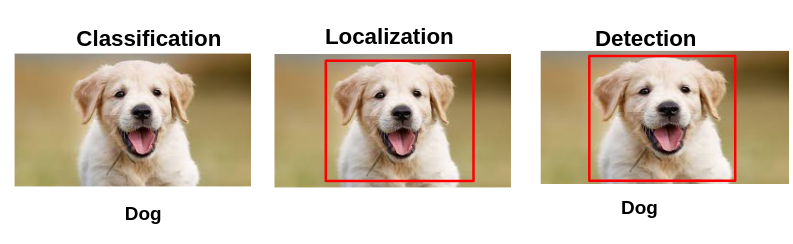So I hope you have a basic understanding of the concept of object detection. In case you want to study object detection in detail, you can read the following blogs-

There are various algorithms for object detection tasks and these algorithms have evolved in the last decade. To improve the performance further, and capture objects of different shapes and sizes, the algorithms predict multiple bounding boxes, of different sizes and aspect ratios.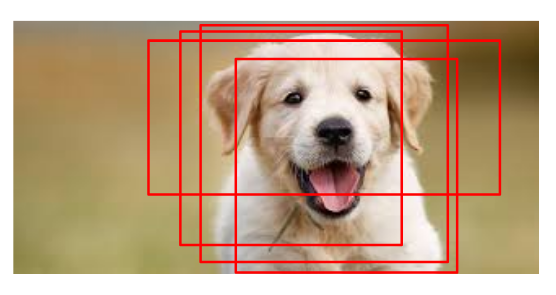But of all the bounding boxes, how is the most appropriate and accurate bounding box selected? This is where NMS comes into the picture.

## What is non-max suppression?

The objects in the image can be of different sizes and shapes, and to capture each of these perfectly, the object detection algorithms create multiple bounding boxes. (left image). Ideally, for each object in the image, we must have a single bounding box. Something like the image on the right.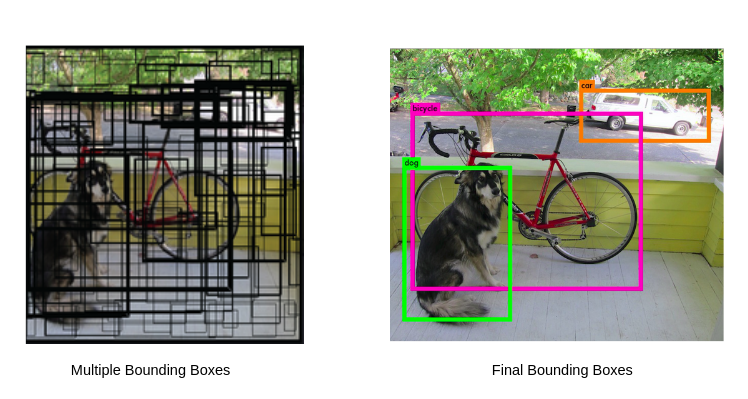Source: https://pjreddie.com/darknet/yolov1/

To select the best bounding box, from the multiple predicted bounding boxes, these object detection algorithms use non-max suppression. This technique is used to “suppress” the less likely bounding boxes and keep only the best one.

So we now understand why do we need NMS and what is it used for. Let us now understand how exactly is the concept implemented.

## How does non-max suppression work?

The purpose of non-max suppression is to select the best bounding box for an object and reject or “suppress” all other bounding boxes. The NMS takes two things into account

1. The objectiveness score is given by the model
2. The overlap or IOU of the bounding boxes

You can see the image below, along with the bounding boxes, the model returns an objectiveness score. This score denotes how certain the model is, that the desired object is present in this bounding box.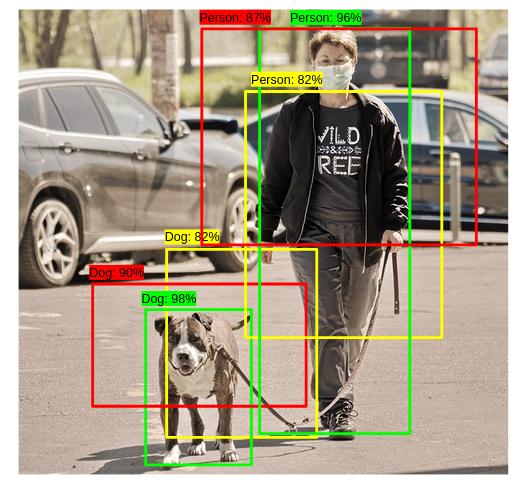You can see all the bounding boxes have the object, but only the green bounding box one is the best bounding box for detecting the object. Now how can we get rid of the other bounding boxes?

The non-max suppression will first select the bounding box with the highest objectiveness score. And then remove all the other boxes with high overlap. So here, in the above image,

1. We will select the Green bounding box for the dog (since it has the highest objectiveness score of 98%)
2. And remove yellow and red boxes for the dog (because they have a high overlap with the green box)

The same process goes for the remaining boxes. This process runs iteratively until there is no more reduction of boxes. In the end, we will be left with the following result.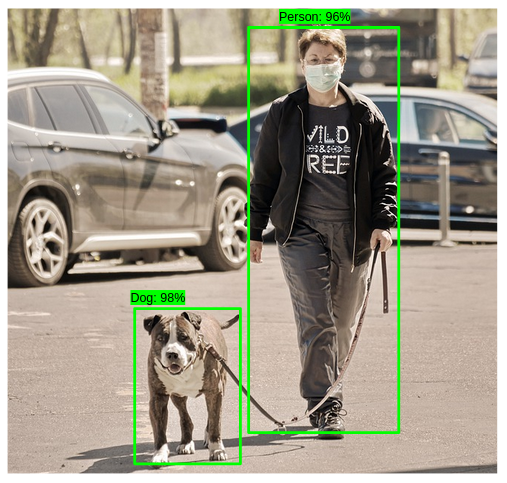That’s it. That’s how NMS works. To solidify our understanding, let’s write a pseudo code to implement non-max suppression.

## Pseudo code for non-max suppression?

By now you would have a good understanding of non-max suppression. Let us break down the process of non-max suppression into steps.

Suppose you built an object detection model to detect the following – Dog or Person. This object detection mode has given the following set of bounding boxes along with the objectiveness scores.The following is the process of selecting the best bounding box using NMS-

Step 1: Select the box with highest objectiveness score

Step 2: Then, compare the overlap (intersection over union) of this box with other boxes

Step 3: Remove the bounding boxes with overlap (intersection over union) >50%

Step 4: Then, move to the next highest objectiveness score

Step 5: Finally, repeat steps 2-4

For our example, this loop will run twice. The below images show the output after different steps.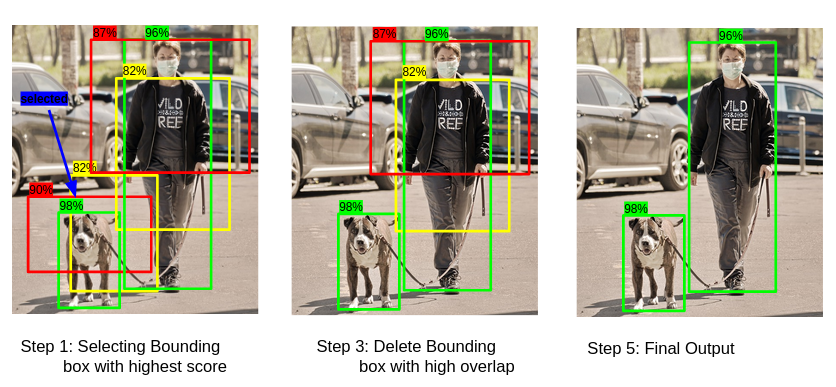## Implementing non-max suppression

Now that you have a good understanding of non-max suppression and how it works, let us look at a simple implementation of the same. Let us say that we have the same image of person and dog (which we have been using in the previous section) with six bounding boxes and the objectiveness score for each of these bounding boxes.

Let us load the image and plot all the six bounding boxes.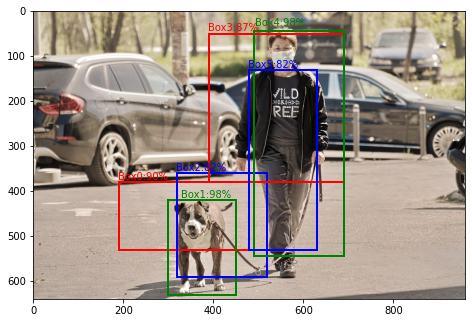For this image, we are going to use the non-max suppression function nms() from the torchvision library. This function requires three parameters-

• Boxes: bounding box coordinates in the x1, y1, x2, y2 format
• Scores: Objectiveness score for each bounding box
• iou_threshold: the threshold for the overlap (or IOU)

Here, since the above coordinates are in x1, y1, width, height format, we will determine the x2, y2 in the following manner-

```x2 = x1 + width
y2 = y1 + height```

#### Output:

`tensor([1, 4])`

So this functions returns the list of bounding box/boxes to keep as an output, in the decreasing order of objectiveness score. Since I have set a very low threshold, the output has only two boxes. But if you set a higher threshold value, you will get more number of bounding boxes. In that case, you can then select the top n bounding boxes (where n should be the number of objects in your image).

For our example, this function has returned the bounding box 1 and 4. Let us plot these on the image to see the final results.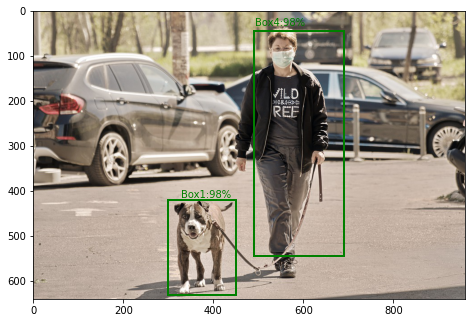Great! So we have our best bounding boxes for each of the object in the image. Now this is a very useful technique and is implemented in most of the object detection algorithms. Let us have a look at some of them in the next section.

## Algorithms that use non-max suppression?

Almost all object detection algorithms use this technique to get the best bounding boxes from the predicted bounding box. The following is the screenshot of the SSD (Single Shot Detector) architecture taken from the research paper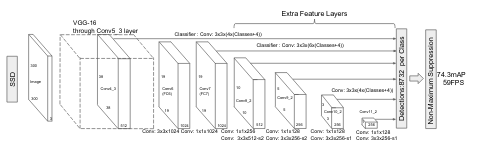You can see that at the final step, SSD has 8732 predicted bounding boxes. Further, after these predictions, SSD uses the non-max suppression technique to select the best bounding box for each object in the image.

Similar to SSD, YOLO (You Only Look Once) also uses non-max suppression at the final step. Multiple bounding boxes are predicted to accommodate objects of different sizes and aspect ratios. Further, from these predictions, NMS to select the best bounding box.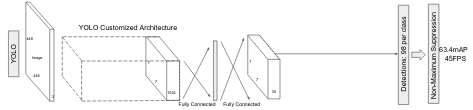## End Notes

To summarize, this article covers the concept of non-max suppression which is an important part of the object detection algorithms. And if you want to explore object detection algorithms, you can check out the following blogs and courses:

I hope this article gave you a good understanding of the topic. In case you have any suggestions/ideas, feel free to share them in the comment section.###### Aishwarya Singh

An avid reader and blogger who loves exploring the endless world of data science and artificial intelligence. Fascinated by the limitless applications of ML and AI; eager to learn and discover the depths of data science.

## 4 thoughts on "Selecting the Right Bounding Box Using Non-Max Suppression (with implementation)"###### RS says:August 03, 2020 at 9:56 pm